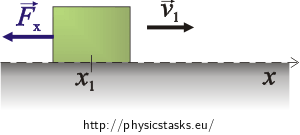## Elastic Force

### Task number: 1996

A rigid body with mass of 2.0 kg is moving on a smooth horizontal surface in the direction of x axis. Resulting force acting on the body has only one non-zero component, the one in the direction of x expressed as Fx = kx, where k = −6 N·m−1. Coordinate x is given in meters. Speed of the body in x1 = 3 m equals v1 = 8.0 m·s−1.

.

a) What is the speed of the body in position x2 = 4 m?

b) In which position has the body speed of v3 = 5.0 m·s−1?• #### Given values

 m = 2,0 kg body mass Fx = kx resulting force acting on the bod k = −6 N·m−1 constant v1 = 8.0 m·s−1 speed of the body at x1 = 3 m v2 = ? m·s−1 speed of the body at x2 = 4 m x3 = ? m position at which the body has speed v3 = 5.0 m·s −1
• #### Analysis

The force acting on the body takes the form of elastic force and it acts against the direction of the movement. You can imagine that the body hits a spring and presses it. Work of elastic force is equal to the change of kinetic energy.

• #### Hint 1 – work of force Fx

What does the force Fx equal when moving from position x1 to position x2. Don’t forget that the force is not constant, it depends on the x coordinate.

• #### HInt 2 – change of kinetic energy

Work of force Fx is equal to the change of kinetic energy of the body. Write an equation for that. Notice if work of Fx is positive or negative and decide, whether kinetic energy of the body and therefore even its speed will grow or diminish.

• #### Hint 3 a) – speed of the body

From equation (2) express the speed v2.

• #### Hint 3 b) – position of the body

Solving b) we use the same idea. The change of kinetic energy when moving from position x1 to position x3 is equal to work of force Fx.

• #### Numerical Solution

a)

$m\,=\,2.0\, \mathrm{kg}$ $k\,=\,\,-\,6\,\mathrm{N \cdot m^{-1}}$ $x_{1}\,=\,3\, \mathrm{m}$ $x_{2}\,=\,4\, \mathrm{m}$ $v_{1}\,=\,8\, \mathrm{m \cdot s^{-1}}$ $v_{2}\,=\,?$
$v_{2}\,=\,\sqrt{v_{1}^{2}\,-\,\frac{k}{m}x_{1}^{2}\,+\,\frac{k}{m}x_{2}^{2}}\,=\,\sqrt{8^{2}\,-\,\frac{\,-\,6}{2}3^{2}\,+\,\frac{\,-\,6}{2}4^{2}}\,\mathrm{m \cdot s^{-1}}$ $v_{2}\,\dot=\,6.56\, \mathrm{m \cdot s^{-1}}$

b)

$m\,=\,2.0\, \mathrm{kg}$ $k\,=\,\,-\,6\,\mathrm{N \cdot m^{-1}}$ $x_{1}\,=\,3\, \mathrm{m}$ $v_{1}\,=\,8\, \mathrm{m \cdot s^{-1}}$ $v_{2}\,=\,5\, \mathrm{m \cdot s^{-1}}$ $x_{3}\,=\,?$
$x_{3}\,=\,\sqrt{\,-\,\frac{m}{k}v_{3}^{2}\,+\,\frac{m}{k}v_{1}^{2}\,+\,x_{1}^{2}}=\,\sqrt{\,-\frac{2}{6}\,5^{2}\,+\,\frac{2}{6}\,8^{2}\,+\,3^{2}}\,\mathrm{m}$ $x_{3}\,\dot=\,4.69\, \mathrm{m}$
• #### Answer

a) Desired velocity is: $v_{2}\,=\,\sqrt{v_{1}^{2}\,-\,\frac{k}{m}x_{1}^{2}\,+\,\frac{k}{m}x_{2}^{2}}\,\dot=\,6.56\, \mathrm{m \cdot s^{-1}}.$

b) Desired position is: $x_{3}\,=\,\sqrt{\frac{m}{k}v_{3}^{2}\,-\,\frac{m}{k}v_{1}^{2}\,+\,x_{1}^{2}}\,\dot=\,4.69\, \mathrm{m}.$

• #### Complete Solution of a)

The force acting on the body takes the form of elastic force and it acts against the direction of the movement. You can imagine that the body hits a spring and presses it. Work of elastic force is equal to the change of kinetic energy.

There is a force acting on the body in the direction of its displacement that is variable and depends on x coordinate. We evaluate this force from formula:

$W\,=\,\int_{x_1}^{x_2}{F_x\mathrm{d}x}\,.$

Then we substitute for force $$F_x\,=\,kx$$ and integrate:

$W\,=\,\int_{x_1}^{x_2}{kx\mathrm{d}x}\,=\,\frac{1}{2}kx_2^2\,-\,\frac{1}{2}kx_1^2\,.\tag{1}$

Work of force Fx is equal to the change of kinetic energy of the body.

$W\,=\,\mathrm{\Delta} E_k\,=\, \frac{1}{2}mv_2^2\,-\,\frac{1}{2}mv_1^2$

Let’s substitute for W from the equation (1).

$\frac{1}{2}kx_2^2\,-\,\frac{1}{2}kx_1^2\,=\,\frac{1}{2}mv_2^2\,-\,\frac{1}{2}mv_1^2\tag{2}$

Work of force Fx is negative – that means it is the body that does work and its kinetic energy and speed are decreasing.

We multiply the equation by 2:

$mv_{2}^{2}\,-\,mv_{1}^{2}\,=\,\,-\,kx_{1}^{2}\,+\,kx_{2}^{2}\,.$

We add the expression $$mv_{1}^{2}$$:

$mv_{2}^{2}\,=\,mv_{1}^{2}\,-\,kx_{1}^{2}\,+\,kx_{2}^{2}\,.$

Then divide by mass m:

$v_{2}^{2}\,=\,v_{1}^{2}\,-\,\frac{k}{m}x_{1}^{2}\,+\,\frac{k}{m}x_{2}^{2}\,.$

We got the second power of speed that we have been looking for. Now let’s just take the square root:

$v_{2}\,=\,\sqrt{v_{1}^{2}\,-\,\frac{k}{m}x_{1}^{2}\,+\,\frac{k}{m}x_{2}^{2}}\,,$ $v_{2}\,=\,\sqrt{8^{2}\,-\,\frac{-6}{2}3^{2}\,+\,\frac{-6}{2}4^{2}}\,\mathrm{m \cdot s^{-1}},$ $v_{2}\,\dot=\,6.56\, \mathrm{m \cdot s^{-1}}.$

Answer: Desired velocity is: $$v_{2}\,\dot=\,6.56\, \mathrm{m \cdot s^{-1}}$$.

• #### Complete Solution of b)

The force acting on the body takes the form of elastic force and it acts against the direction of the movement. You can imagine that the body hits a spring and presses it. Work of elastic force is equal to the change of kinetic energy.

There is a force acting on the body in the direction of its displacement that is variable and depends on x coordinate. We evaluate this force from formula:

$W\,=\,\int_{x_1}^{x_3}{F_x\mathrm{d}x}.$

Then we substitute for force $$F_x\,=\,kx$$ and integrate:

$W\,=\,\int_{x_1}^{x_3}{kx\mathrm{d}x}\,=\,\frac{1}{2}kx_3^2\,-\,\frac{1}{2}kx_1^2.\tag{1}$

Work of elastic force is equal to the change of kinetic energy:

$W\,=\,\Delta E_k\,=\, \frac{1}{2}mv_3^2\,-\,\frac{1}{2}mv_1^2.$

Let’s substitute for W from the equation (1).

$\frac{1}{2}kx_3^2\,-\,\frac{1}{2}kx_1^2\,=\,\frac{1}{2}mv_3^2\,-\,\frac{1}{2}mv_1^2.\tag{2}$

From that we express the desired position x3. We multiply the equation by 2:

$mv_{3}^{2}\,-\,mv_{1}^{2}\,=\,kx_{3}^{2}\,-\,kx_{1}^{2}.$

We add the expression $$kx_{1}^{2}$$:

$kx_{3}^{2}\,=\,mv_{3}^{2}\,-\,mv_{1}^{2}\,+\,kx_{1}^{2}.$

Then divide by k:

$x_{3}^{2}\,=\,\frac{m}{k}v_{3}^{2}\,-\,\frac{m}{k}v_{1}^{2}\,+\,x_{1}^{2}.$

We got the second power of speed that we have been looking for. Now let’s just take the square root.

$x_{3}\,=\,\sqrt{\frac{m}{k}v_{3}^{2}\,-\,\frac{m}{k}v_{1}^{2}\,+\,x_{1}^{2}},$ $x_{3}\,=\,\sqrt{-\frac{2}{6}5^{2}\,+\,\frac{2}{6}8^{2}\,+\,3^{2}}\,\mathrm{m},$ $x_{3}\,=\,4.69\, \mathrm{m}.$

Odpověď: Desired position is:$$\,x_{3}\,\dot=\,4.69\, \mathrm{m}$$.×Original source: Halliday,D., Resnick,R., Walker,J.: Fyzika. Vysokoškolská učebnice obecné fyziky. VUTIUM, Brno 2000
Zpracováno v bakalářské práci Jany Šimkové (2008).